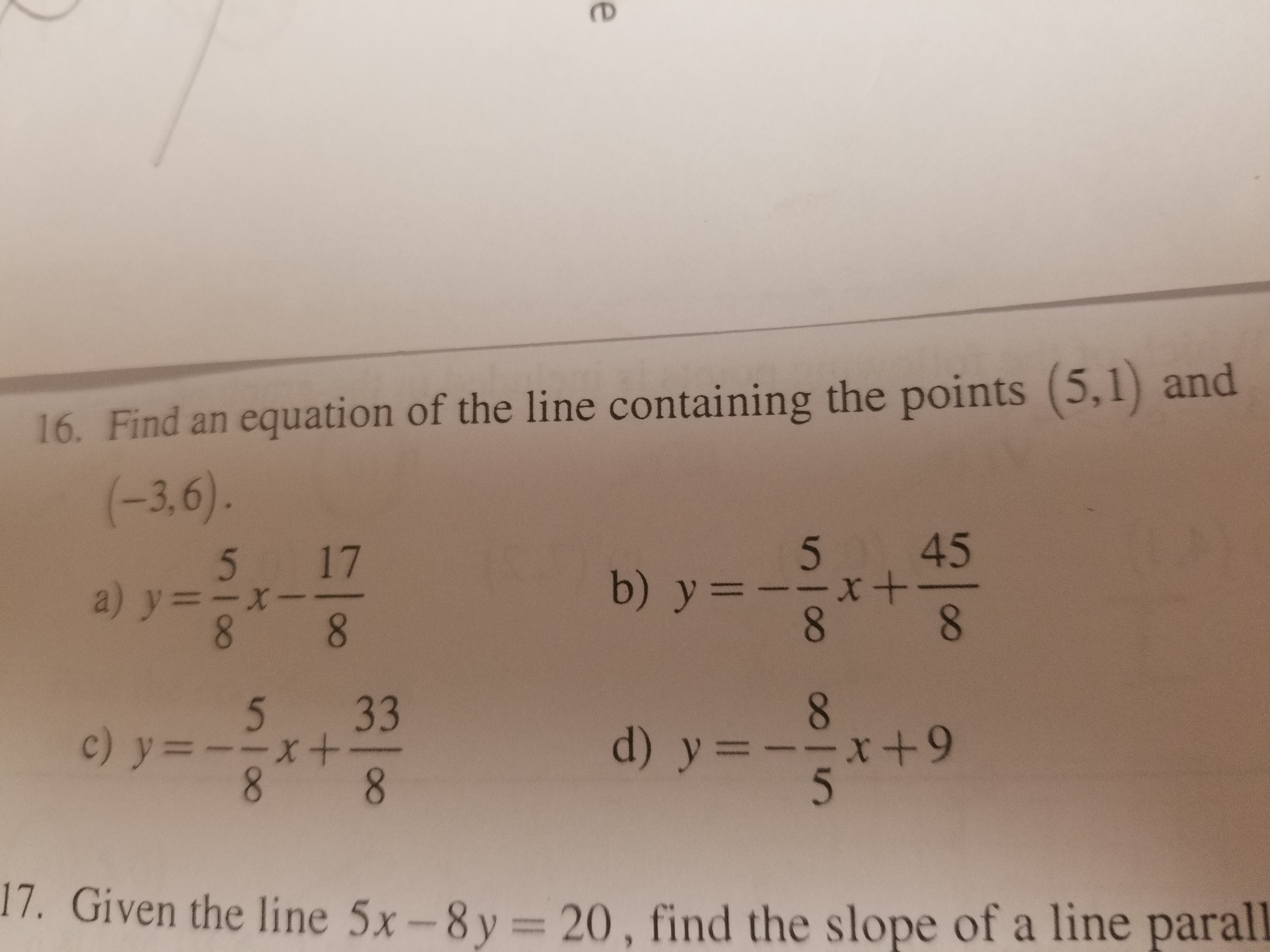# 16. Find an equation of the line containing the points (5.1) and5 175 45ㄧㄧㄧㄨ5 3317. Given the line 5x -8y20, find the slope of a line parall

Question
6 viewshelp_outlineImage Transcriptionclose16. Find an equation of the line containing the points (5.1) and 5 17 5 45 ㄧㄧㄧㄨ 5 33 17. Given the line 5x -8y 20, find the slope of a line parall fullscreen
check_circle

Step 1

Equation of a line containing the points (x1,y1) and (x2,y2) is given by the equation

Here,

Plugging the values in the equation,

Therefore, the equatio...

### Want to see the full answer?

See Solution

#### Want to see this answer and more?

Solutions are written by subject experts who are available 24/7. Questions are typically answered within 1 hour.*

See Solution
*Response times may vary by subject and question.
Tagged in

### Algebra